# 基于Matlab的数字水印设计—基于DCT域的水印算法实现

1 设计目的

2 设计要求

3 数字水印技术基本原理

3.1 数字水印基本框架

3.2 算法分类

3.2.1 DCT法

3.2.2 其他方法

3.3 实际需要考虑的问题

3.3.1 不可见性

3.3.2 鲁棒性

3.3.3 水印容量

3.3.4 安全性

4 基于DCT变换仿真

4.1 算法原理

4.1.1 准备工作

4.1.2 选取8*8变换块

4.1.3 边界自适应

4.1.4 DCT变换与嵌入

4.1.5 恢复空域8

4.2 嵌入算法扩展

4.2.1 RGB彩色图像三个矩阵的划分

4.2.2 八色彩色水印

4.3 水印的提取

4.4 仿真程序

5 结果分析4

3.1 数字水印基本框架

3.2 算法分类

3.2.1 DCT法

3．3 实际需要考虑的问题

3.3.1 不可见性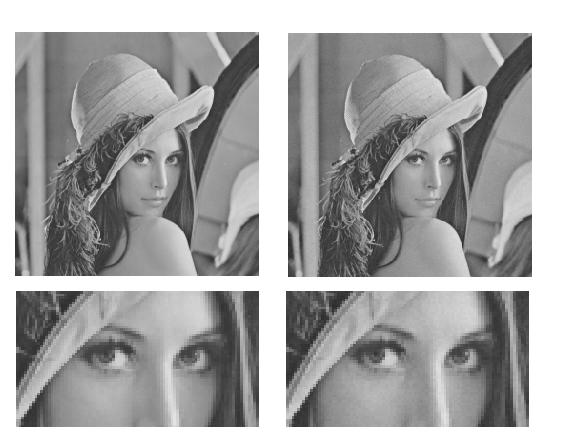3.3.2 鲁棒性

3.3.3 水印容量

3.3.4 安全性

4.1 算法原理

DCT变换域数字水印算法的基本原理是将空域图像变成频域，然后将水印信息嵌入其直流项之中，最后将频域转换成空域以完成图片的水印的嵌入。其主要思想是：在DCT变换域上选择中、低频系数叠加水印信息，因为人眼的感觉主要集中在中、低频段，攻击者破坏水印时，不可避免地会引起图像质量的严重下降，而且一般的图像处理也不会改变这部分数据。再者，由于JPEG、MPEG等压缩算法的核心是在DCT变换域上进行量化，故通过巧妙的融合水印和量化过程，可以使水印抵御一定的有损压缩。此外，DCT变换域系数的统计分布有比较好的数学模型，可以从理论上估计水印的信息量。基于DCT变换的数字水印在逆变换时会散布在整个图像空间中，故水印不像空间域技术那样易受到裁剪、低通滤波等攻击的影响，具有鲁棒性高、隐蔽性好的特点。

4.1.1 DCT变换与嵌入

4.1.2 恢复空域

4.2 嵌入算法扩展

4.2.1 RGB彩色图像三个矩阵的划分

1、彩色图像的边缘图像

2、彩色图像分层

4.2.2 八色彩色水印

4.3 水印的提取

4.4 源码

blockno=size/block;   %每行块的个数
LENGTH=size*size/64;   %总块的个数1024
Alpha1=0.03;   %非边界处的强度因子
Alpha2=0.1;    %边界处的强度因子
T1=3;   %域值为三个边界点
I=zeros(size,size);   %原始图像
BW=zeros(size,size);   %原始图像的边缘图
block_dct1=zeros(block,block);
mark1=logical(mark(:,:,1));
mark2=logical(mark(:,:,2));
mark3=logical(mark(:,:,3));
figure(1)
subplot(2,2,1);
imshow(mark);title('水印图像');
%显示原图
imshow(I);title('原始图像:I');
%显示prewitt为算子的边缘图
GRAY=rgb2gray(I);   %变灰度要用三位数，即彩色图片
BW=edge(GRAY,'prewitt');   %取边界，用于边界自适应
subplot(2,2,3);imshow(BW);
title('原始图像的边缘');
%嵌入水印
for m=1:blockno;   %到第（m,n）个块
for n=1:blockno;
x=(m-1)*block+1;   %该块的起始像素
y=(n-1)*block+1;
block_dct1=I(x:x+block-1,y:y+block-1);   %取该块所有像素
block_dct1=dct2(block_dct1);   %变换
%第二维
block_dct2=I(x:x+block-1,y:y+block-1,2);   %取该块所有像素
block_dct2=dct2(block_dct2);   %变换
BW_8_8=BW(x:x+block-1,y:y+block-1);   %取边缘的对应块像素
if m<=1|n<=1;%m或n小于等于1时，
T=0;
else
T=sum(BW_8_8);   %列取和
T=sum(T);   %对整个BW_8_8中的数字取和
%取和
end
if T>T1;   %如果改为<则意味着高频区嵌入了低能量，低频区嵌入了高能量。所以在低频区嵌入的过高能量会是不该看出的水印显示出来
%因此这里表示着边缘自适应性
Alpha=Alpha2;   %0.1
else
Alpha=Alpha1;   %0.03
end
block_dct1(1,1)=block_dct1(1,1)*(1+Alpha*(mark1(m,n)-1));   %对直流进行嵌入
block_dct2(1,1)=block_dct2(1,1)*(1+Alpha*(mark2(m,n)-1));   %第二层
block_dct3(1,1)=block_dct3(1,1)*(1+Alpha*(mark3(m,n)-1));   %第三层
block_dct1=idct2(block_dct1);   %变回空域
block_dct2=idct2(block_dct2);   %第二层
block_dct3=idct2(block_dct3);   %第三层
I(x:x+block-1,y:y+block-1)=block_dct1;    %贴回原图像
I(x:x+block-1,y:y+block-1,2)=block_dct2;    %第二层
I(x:x+block-1,y:y+block-1,3)=block_dct3;   %第三层
end
end
%显示嵌入水印后的图像
subplot(2,2,4);imshow(I);title('嵌入水印后的图像');
imwrite(I,'D:\输出图.bmp','bmp');
figure(2);subplot(2,2,1);imshow(mark(:,:,1));title('原水印的第一层');
subplot(2,2,2);imshow(mark(:,:,2));title('原水印的第二层');
subplot(2,2,3);imshow(mark(:,:,3));title('原水印的第三层');
imwrite(I,'D:\压缩图.jpg','jpg','quality',90);   %压缩
%通过原图片对比提取水印
K=zeros(32,32,3);   %水印图片为32*32
for q=1:blockno;
for p=1:blockno;
x=(p-1)*block+1;
y=(q-1)*block+1;
BLOCK1=I(x:x+block-1,y:y+block-1);   %取无水印图的块
BLOCK2=J(x:x+block-1,y:y+block-1);   %取带水印图的块
BLOCK1=dct2(BLOCK1);
BLOCK2=dct2(BLOCK2);
a=BLOCK2(1,1)/BLOCK1(1,1)-1;
%第二层
BLOCK12=I(x:x+block-1,y:y+block-1,2);
BLOCK22=J(x:x+block-1,y:y+block-1,2);
BLOCK12=dct2(BLOCK12);
BLOCK22=dct2(BLOCK22);
b=BLOCK22(1,1)/BLOCK12(1,1)-1;
%第三层
BLOCK13=I(x:x+block-1,y:y+block-1,3);
BLOCK23=J(x:x+block-1,y:y+block-1,3);
BLOCK13=dct2(BLOCK13);
BLOCK23=dct2(BLOCK23);
c=BLOCK23(1,1)/BLOCK13(1,1)-1;
if a<0
W(p,q)=0;   %恢复水印图像
else
W(p,q)=1;
end
if b<0
W2(p,q)=0;
else
W2(p,q)=1;
end
end
end
figure(3);
subplot(2,2,1);imshow(W);
title('从第一层提取的水印（红R）');   %0表示黑色
subplot(2,2,2);imshow(W2);
title('从第二层提取的水印（绿G）');
subplot(2,2,3);imshow(W3);
title('从第三层提取的水印（蓝B）');
K(:,:,1)=W;
K(:,:,2)=W2;
K(:,:,3)=W3;
subplot(2,2,4);imshow(K);title('合并的彩图');
%检测压缩后的水印图像
for q=1:blockno;
for p=1:blockno;
x=(p-1)*block+1;
y=(q-1)*block+1;
BLOCK1=I(x:x+block-1,y:y+block-1);   %取无水印图的块
BLOCK2=J(x:x+block-1,y:y+block-1);   %取带水印图的块
%第二层
BLOCK12=I(x:x+block-1,y:y+block-1,2);
BLOCK22=J(x:x+block-1,y:y+block-1,2);
BLOCK12=dct2(BLOCK12);
BLOCK22=dct2(BLOCK22);
b=BLOCK22(1,1)/BLOCK12(1,1)-1;
%第三层
BLOCK13=I(x:x+block-1,y:y+block-1,3);
BLOCK23=J(x:x+block-1,y:y+block-1,3);
BLOCK13=dct2(BLOCK13);
BLOCK23=dct2(BLOCK23);
c=BLOCK23(1,1)/BLOCK13(1,1)-1;
if a<0
W(p,q)=0;
else
W(p,q)=1;
end
if b<0
W2(p,q)=0;
else
W2(p,q)=1;
end
if c<0
W3(p,q)=0;
else
W3(p,q)=1;
end
end
end
K(:,:,1)=W;K(:,:,2)=W2;K(:,:,3)=W3;
figure(4);imshow(K);title('90压缩的提取');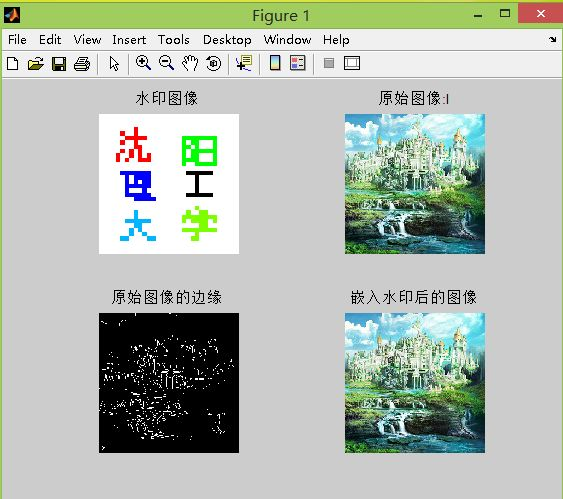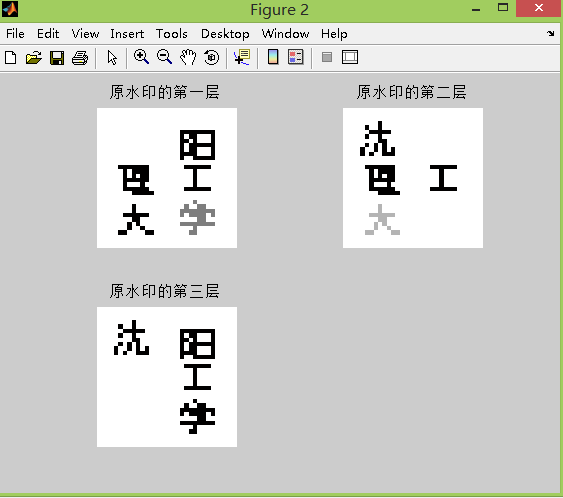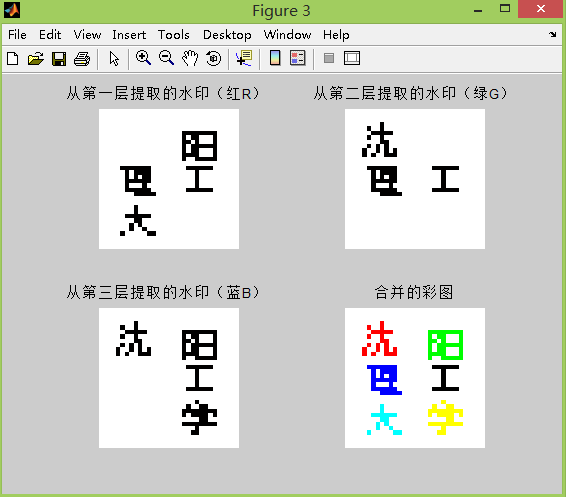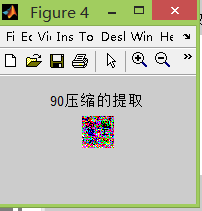阮秋琦. 数字图像处理学[M]. 北京：电子工业出版社，2001.

 龚声蓉，刘纯平，王强等. 数字图像处理与分析[M]. 北京：清华大学出版社，2006.

 贾永红. 计算机图像处理与分析[M]. 武汉：武汉大学出版社，2001.

 陈桂明. 应用MATLAB语言处理数字信号与图像处理[M]. 北京：科学出版社，2000.

 夏德深，傅德胜. 计算机图像处理及应用[M]. 南京：东南大学出版社，2004.

 姚敏. 计算机图像处理[M]. 北京：机械工业出版社，2006.

 容观澳. 计算机图像处理[M]. 北京：清华大学出版社，2000.

 吴健康. 数字图像分析[M]. 北京：邮电出版社，1989.

05-153万+
04-02356
12-151万+
12-241682
05-096468
08-103575
11-192551
02-121460
08-18787
08-16537
08-191799
03-19474
02-12770
09-01882
11-217646

### “相关推荐”对你有帮助么？

•非常没帮助
•没帮助
•一般
•有帮助
•非常有帮助TuTu998

¥2 ¥4 ¥6 ¥10 ¥20余额支付 (余额：-- )扫码支付获取中扫码支付点击重新获取扫码支付1.余额是钱包充值的虚拟货币，按照1:1的比例进行支付金额的抵扣。
2.余额无法直接购买下载，可以购买VIP、C币套餐、付费专栏及课程。余额充值